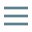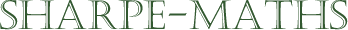Previous topic:
Current topic:
Multiplying fractions
Next topic:
Dividing fractions

### Multiplying fractions

Interestingly, multiplying fractions is, in many ways, simpler than adding or subtracting them, though it is important to learn the method correctly and precisely at otherwise you will end up with a mess.

It is vital to make sure that you work in the correct order.

1. Cancel where possible.
2. Multiply the numerators and denominators together.
3. Check that the fraction can be simplified (if you missed a cancellation in point 1).